# Epimorphism

(diff) ← Older revision | Latest revision (diff) | Newer revision → (diff)

A concept reflecting the algebraic properties of surjective mappings of sets. A morphism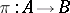in a category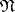is called an epimorphism if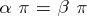implies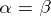. In other words, an epimorphism is a morphism that can be cancelled on the right.

Every isomorphism is an epimorphism. The product of two epimorphisms is an epimorphism. Therefore, all epimorphisms of a categoryform a subcategory of(denoted by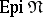).

In the categories of sets, vector spaces, groups, and Abelian groups, the epimorphisms are precisely the surjective mappings, i.e. the linear mappings and the homomorphisms of one set, vector space or group onto another set, vector space or group. However, in the categories of topological spaces or associative rings there are non-surjective epimorphisms (that is, mappings that are not "onto" ).

The concept of an epimorphism is dual to that of a monomorphism.

In the article above,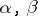are assumed to be morphisms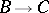for some. If composition of morphism is written from left to right, as is sometimes done, so that the composite ofand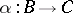is written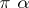, then epimorphisms are of course morphisms that cancel on the left.
The inclusion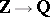in the category of rings is an example of an epimorphism that is not surjective.